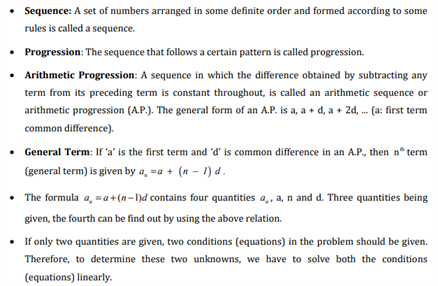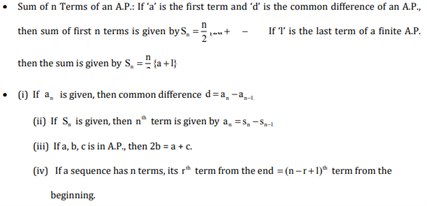# Revision Notes For CBSE Class 10 Maths Chapter 5 Arithmetic Progressions

In general, an arithmetic progression is defined as a sequence of numbers where the difference between any two subsequent numbers is a constant. While it may sound confusing to students, this topic has been broadly discussed in class 10 maths textbook chapter 5. The chapter covers all aspects related to this topic and helps students understand the core concepts like common difference, nth term of an AP, etc. along with suitable examples. The chapter also talks about the right method to find the sum of arithmetic progressions. Keeping in mind the same objective, free CBSE revision notes for class 10 maths chapter 5 – arithmetic progressions is available here for students. They can easily download these notes and use them as a reference tool to boost their productivity and improve their overall math skills. Furthermore, students can use these to gain a clear insight into the chapter and also have an effective revision before the exams.

Students can go through class 10 maths chapter 5 CBSE revision notes PDF below.Access CBSE Class 10 Maths Sample Papers Here.

Access NCERT Class 10 Maths Book Here.